# Chemistry Topic-Wise Test - 7

## 45 Questions MCQ Test NEET Mock Test Series & Past Year Papers | Chemistry Topic-Wise Test - 7

Description
This mock test of Chemistry Topic-Wise Test - 7 for NEET helps you for every NEET entrance exam. This contains 45 Multiple Choice Questions for NEET Chemistry Topic-Wise Test - 7 (mcq) to study with solutions a complete question bank. The solved questions answers in this Chemistry Topic-Wise Test - 7 quiz give you a good mix of easy questions and tough questions. NEET students definitely take this Chemistry Topic-Wise Test - 7 exercise for a better result in the exam. You can find other Chemistry Topic-Wise Test - 7 extra questions, long questions & short questions for NEET on EduRev as well by searching above.
QUESTION: 1

Solution:
QUESTION: 2

Solution:
QUESTION: 3

### Night-blindness may develop due to the defciency of

Solution:
QUESTION: 4
Which one among the following is the most powerfull oxidizing agent ?
Solution:
QUESTION: 5
The colour of bromine (liquid) is
Solution:
QUESTION: 6
Van der Waals force is the strongest in
Solution:
QUESTION: 7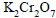reacts with concetrated HCl to produce
Solution:
QUESTION: 8
The structure of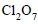is repesented by
Solution:
QUESTION: 9
The noble gas which was discovered first in the sun and then on the earth is
Solution:
QUESTION: 10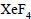is prepared by heating Xe and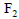at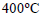in the ratio of

Solution:
QUESTION: 11reacts violently with water to give
Solution:
QUESTION: 12

Xenon trioxide (XeO3) has a

Solution:
QUESTION: 13
For breathing, deep-sea divers use a mixture of
Solution:
QUESTION: 14
Argon was discovered by
Solution:
QUESTION: 15
Chlorampehnicol is used as an
Solution:
QUESTION: 16
Phenol is commonly used as
Solution:
QUESTION: 17
Which of the following is a direct dye for cotton ?
Solution:
QUESTION: 18
Which of the following is used as an oxidizer of rocket fuels ?
Solution:
QUESTION: 19
Which of the following is not a natural polymer ?
Solution:
QUESTION: 20
Natural rubber is a polymer of
Solution:
QUESTION: 21
Orlon in obtained by the polymerization of
Solution:
QUESTION: 22
The catalyst used in the manufacture of polythene by Ziegler-Natta process is
Solution:
QUESTION: 23
Bunna–S rubber (SBR) is a copolymer of
Solution:
QUESTION: 24
The polymer obtained by condensation polymerization is
Solution:
QUESTION: 25
Cellulose is a condensation polymer of
Solution:
QUESTION: 26
Glucose and fructose are
Solution:
QUESTION: 27
Starch consists of two polymeric units, namely
Solution:
QUESTION: 28
The colour of the precipitate formed when a reducing sugar is heated with Fehling’s solution is
Solution:
QUESTION: 29
Which of the following is an amino acids ?
Solution:
QUESTION: 30
Identify the functional group present in the dipeptide formed from alanine only ?
Solution:
QUESTION: 31
The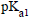and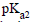vlaues of alanine are 2.3 and 9.7 respectively. The isoelectric point of alanine is
Solution:
QUESTION: 32
The portions of proteins having the highest mobility are
Solution:
QUESTION: 33
Nucleic acids are called acids mainly because of the presence of
Solution:
QUESTION: 34
The value of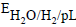at 298K would be
Solution:
QUESTION: 35
How much will the potential of a hydrogen electrode change when its solution initially at pH = 0 is neutralised to pH = 7 ?
Solution:
QUESTION: 36
Equal quantities of electricity are passed through three voltmeters containing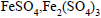and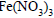consider the following statements in this regard
I.The amount of iron deposited in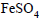and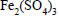II.The amount of iron deposited inis two third of teh amount of iron deposited inIII.The amount of iron deposited in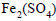andis equal to
Solution:
QUESTION: 37
The volume of the gas liberated at STP when a current of
5.36 amp is passed through dil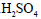for 5 hour will be
Solution:
QUESTION: 38
On electrolysis, which of the following does not give out oxygen ?
Solution:
QUESTION: 39
The standard reduction potentials at 298 K for the half reactions are given against each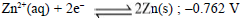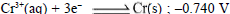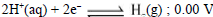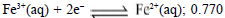Which is the strongest reducing agent ?
Solution:
QUESTION: 40
From the following  values of half cells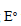values of half cells
(i)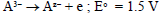(ii)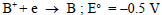(iii)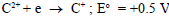(iv)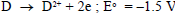What combination of two half cells would result in a cell with the largest potential ?
Solution:
QUESTION: 41
The chemical reaction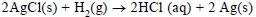taking place is a galvanic cell is represented by the rotation
Solution:
QUESTION: 42
The e.m.f. of the cell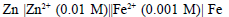at 298 K is 0.02905 volt. The the value of equilibrium constant for the cell reaction is
Solution:
QUESTION: 43
The standard reduction potentials for two reactions are given below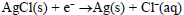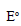= 0.22 V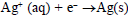= 0.80 V
The solubility product of AgCl under standard condtions of temperature (298 K) is given by
Solution:
QUESTION: 44
The half cell reactions for rusting of iron are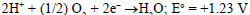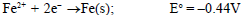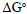(in KJ) for the reaction is
Solution:
QUESTION: 45
240 couloumbs of electricity is passed through a solution of dilute sulphuric acid for 20 minutes. The number of amperes of current produced is
Solution: